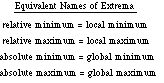index: click on a letter A B C D E F G H I J K L M N O P Q R S T U V W X Y Z A to Z index index: subject areas numbers & symbols sets, logic, proofs geometry algebra trigonometry advanced algebra & pre-calculus calculus advanced topics probability & statistics real world applications multimedia entrieswww.mathwords.com about mathwords website feedback

 Extremum An extreme value of a function. In other words, the minima and maxima of a function. Extrema may be either relative (local) or absolute (global). Note: The first derivative test and the second derivative test are common methods used to find extrema.See also